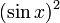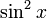# Sine-squared function

(diff) ← Older revision | Latest revision (diff) | Newer revision → (diff)

## Definition

This function, denoted$\sin^2$, is defined as the composite of the square function and the sine function. Explicitly, it is the map:$x \mapsto (\sin x)^2$

For brevity, we write$(\sin x)^2$ as$\sin^2x$.

## Key data

Item Value
Default domain all real numbers, i.e., all of$\R$
range$[0,1]$, i.e.,$\{ y \mid 0 \le y \le 1 \}$
period$\pi$, i.e.,$180\,^\circ$
local maximum value and points of attainment All local maximum values are equal to 1, and are attained at odd integer multiples of$\pi/2$.
local minimum value and points of attainment All local minimum values are equal to 0, and are attained at integer multiples of$\pi$.
points of inflection (both coordinates) odd multiples of$\pi/4$, with value 1/2 at each point.
derivative$x \mapsto \sin(2x) = 2\sin x \cos x$, i.e., double-angle sine function.
antiderivative$x \mapsto \frac{x}{2} - \frac{\sin(2x)}{4} + C$
mean value over a period 1/2
expression as a sinusoidal function plus a constant function$(1/2) - \cos(2x)/2$